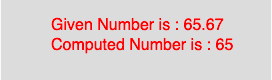# p5.js | floor() function

• Last Updated : 09 Apr, 2019

The floor() function in p5.js is used to calculate the floor value of a number. This function maps to the Math.floor() of javascript. It calculates the closest int value that is less than or equal to the value of the parameter.

Syntax

```floor(number)
```

Parameters: The function accepts only one parameter as mentioned above and described below:

• number : This parameter stores the number to compute.
• Below program illustrates the floor() function in p5.js:
Example:

 `function` `setup() { ` ` `  `    ``//create Canvas of size 270*80   ` `    ``createCanvas(270, 80); ` `} ` ` `  `function` `draw() { ` `    ``background(220); ` `    ``//initialize the parameter   ` `    ``let x = 65.67; ` `    ``//call to floor() function   ` `    ``let y = floor(x); ` `    ``textSize(16); ` `    ``fill(color(``'red'``)); ` `    ``text(``"Given Number is : "` `+ x, 50, 30); ` `    ``text(``"Computed Number is : "` `+ y, 50, 50); ` `} `

Output:Reference: https://p5js.org/reference/#/p5/floor

My Personal Notes arrow_drop_up
Recommended Articles
Page :